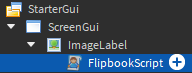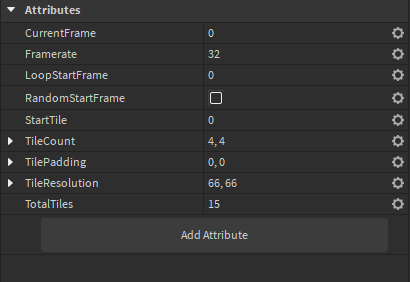# Flipbook animation script for ImageLabels or Buttons

Copy and paste the following code into a LocalScript that is a child of an ImageLabel or ImageButton.

(or you can just insert this model: Flipbook Script - Roblox)

``````-- Created by Noobot9k

if script.Parent and (script.Parent:IsA("ImageLabel") or script.Parent:IsA("ImageButton")) then else error(script:GetFullName().. " must be a child of a ImageLabel or ImageButton.") end

local RunService = game:GetService("RunService")

local CurrentFrame = 0
local _nextFrame = tick()
local itterateConnection : RBXScriptConnection?

if script:GetAttribute("RandomStartFrame") then
local TileCount : Vector2 = script:GetAttribute("TileCount")
script:SetAttribute("CurrentFrame", math.random(0, TileCount.X * TileCount.Y))
end

function itterate(_, deltaTime)

local Framerate : number =			script:GetAttribute("Framerate")
local LoopStartFrame : number =		script:GetAttribute("LoopStartFrame") or 0
local TileCount : Vector2 =			script:GetAttribute("TileCount")
local TotalTiles : number =			script:GetAttribute("TotalTiles") or TileCount.X * TileCount.Y
local StartTile : number =			script:GetAttribute("StartTile") or 0
local TileResolution : Vector2 =	script:GetAttribute("TileResolution")
CurrentFrame = 						math.max(script:GetAttribute("CurrentFrame"), StartTile)

local Y = math.floor(CurrentFrame / TileCount.X)
local X = CurrentFrame - (Y * TileCount.X)

script.Parent.ImageRectSize = TileResolution
script.Parent.ImageRectOffset = (TileResolution + TilePadding) * Vector2.new(X, Y)

if tick() >= _nextFrame then
_nextFrame = tick() + (1 / Framerate)
CurrentFrame = CurrentFrame + 1
end
if CurrentFrame >= TotalTiles then CurrentFrame = math.max(LoopStartFrame, StartTile) end -- CurrentFrame >= TileCount.X * TileCount.Y
if script:GetAttribute("CurrentFrame") ~= CurrentFrame then script:SetAttribute("CurrentFrame", CurrentFrame) end

end
function disable()
if itterateConnection then itterateConnection:Disconnect() itterateConnection = nil end
end
function enable()
disable()
itterateConnection = RunService.Stepped:Connect(itterate)
end
enable()
``````

Your hierarchy should look something like this when you’re done:Add the following attributes to the LocalScript:`CurrentFrame`, `Framerate`, `LoopStartFrame`, `StartTile`, and `TotalTiles` are numbers.
`RandomStartFrame` is a boolean.
`TileCount`, `TilePadding`, and `TileResolution` are all Vector2s.

Set the image of the ImageLabel or ImageButton to be one with a grid of smaller images on it that are each similar looking but slightly different.

Set the TileCount attribute to be how many smaller images are in the larger image along the X-axis and the Y-axis. X is how many columns and Y is how many rows.

Set the TileResolution to the resolution of each tile in the source image. If your image is 1024x1024 and your TileCount is 4x4 then your TileResolution should be 256x256.
Note that the max image resolution on Roblox is 1024x1024 so if you upload a 2048x2048 image with a TileCount of 4x4, your TileResolution wouldn’t be 512x512, it would still be 256x256 as Roblox would downscale your texture to 1024².

Set TotalTiles to the total number of tiles in your animation across all rows and columns. If TileCount is 4x4 but the bottom row only has 3 images and then an empty space, then you should set TotalTiles to 15 instead of 16.

The Framerate attribute is how fast your animation plays. Higher values are faster and lower ones are slower. 16 is usually a good starting value.

If this animation isn’t always visible, it would be a good idea to make another script disable this one to save on performance so it’s not running in the background. Roblox unfortunately doesn’t provide an easy way to check if a UI item is visible or not.

See my reply below for an example of it in action.

11 Likes

Not exactly sure what you mean by “flipbook animation”, since alone it’s pretty vague on what it could look like. Perhaps a video or gif showing it could help?

Here’s an example:

This flipbook tileset image is 896x896.

Its TileCount would be 7x7.
TileResolution would be 128x128.
Framerate should be 60 in this case.
TotalTiles should be 49 and not 50 since there’s an empty spot in the bottom-right corner.

In-game, it would look something like this:You can use this website to preview what your flipbook animation will look like before you upload it to Roblox:
https://is.si/animator/

This is mostly meant to be used for UI items like a saving or loading animation.

1 Like

You can make Never Gonna with that lmao

1 Like

What programm can i use to generate such a flipbook image. For example from a video

If you make the animation with Blender you can render each frame out as its own PNG and then use a program to combine them into a single tileset like the example I gave above. This is the program I use.

If you have a video you’d like to do this with then you’ll need to find a program that can split it into individual PNG files of each frame.